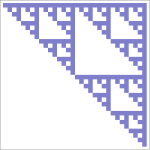## 7.7 Sobol’ Sampler

The last Sampler in this chapter is based on a series of generator matrices due to Sobol. The samples from the sequence that these matrices generate are distinguished by both being very efficient to implement—thanks to being entirely based on base-2 computations—while also being extremely well distributed over all dimensions of the sample vector. Figure 7.34 shows the first few Sobol generator matrices.Figure 7.35 compares Sobol samples to stratified and Halton points with the depth of field test scene.

The weakness of the Sobol points is that they are prone to structural grid artifacts before convergence; a sense of this issue can be seen in the image sample points shown in Figure 7.36.

This structure is visible in the images in Figure 7.37. In exchange for this weakness, Sobol sequences are extremely well distributed over all dimensions of the sample vector.

<<SobolSampler Declarations>>=
class SobolSampler : public GlobalSampler { public: <<SobolSampler Public Methods>>
std::unique_ptr<Sampler> Clone(int seed); SobolSampler(int64_t samplesPerPixel, const Bounds2i &sampleBounds) : GlobalSampler(RoundUpPow2(samplesPerPixel)), sampleBounds(sampleBounds) { resolution = RoundUpPow2(std::max(sampleBounds.Diagonal().x, sampleBounds.Diagonal().y)); log2Resolution = Log2Int(resolution); } int64_t GetIndexForSample(int64_t sampleNum) const; Float SampleDimension(int64_t index, int dimension) const;
private: <<SobolSampler Private Data>>
const Bounds2i sampleBounds; int resolution, log2Resolution;
};

The SobolSampler uniformly scales the first two dimensions by the smallest power of 2 that causes the sample domain to cover the image area to be sampled. As with the HaltonSampler, this specific scaling scheme is chosen in order to make it easier to compute the reverse mapping from pixel coordinates to the sample indices that land in each pixel.

<<SobolSampler Public Methods>>=
SobolSampler(int64_t samplesPerPixel, const Bounds2i &sampleBounds) : GlobalSampler(RoundUpPow2(samplesPerPixel)), sampleBounds(sampleBounds) { resolution = RoundUpPow2(std::max(sampleBounds.Diagonal().x, sampleBounds.Diagonal().y)); log2Resolution = Log2Int(resolution); }

<<SobolSampler Private Data>>=
const Bounds2i sampleBounds; int resolution, log2Resolution;

The SobolIntervalToIndex() function returns the index of the sampleNumth sample in the pixel p, if the sampling domain has been scaled by to cover the pixel sampling area.

<<Low Discrepancy Declarations>>+=
inline uint64_t SobolIntervalToIndex(const uint32_t log2Resolution, uint64_t sampleNum, const Point2i &p);

The general approach used to derive the algorithm it implements is similar to that used by the Halton sampler in its GetIndexForSample() method. Here, scaling by a power of two means that the base-2 logarithm of the scale gives the number of digits of the product that form the scaled sample’s integer component. To find the values of that give a particular integer value after scaling, we can compute the inverse of : given

then equivalently

We won’t include the implementation of this method here.

<<SobolSampler Method Definitions>>=
int64_t SobolSampler::GetIndexForSample(int64_t sampleNum) const { return SobolIntervalToIndex(log2Resolution, sampleNum, Point2i(currentPixel - sampleBounds.pMin)); }

Computing the sample value for a given sample index and dimension is straightforward given the SobolSample() function.

<<SobolSampler Method Definitions>>+=
Float SobolSampler::SampleDimension(int64_t index, int dim) const { Float s = SobolSample(index, dim); <<Remap Sobol dimensions used for pixel samples>>
if (dim == 0 || dim == 1) { s = s * resolution + sampleBounds.pMin[dim]; s = Clamp(s - currentPixel[dim], (Float)0, OneMinusEpsilon); }
return s; }

The code for computing Sobol sample values takes different paths for 32- and 64-bit floating-point values. Different generator matrices are used for these two cases, giving more bits of precision for 64-bit doubles.

<<Low Discrepancy Inline Functions>>+=
inline Float SobolSample(int64_t index, int dimension, uint64_t scramble = 0) { #ifdef PBRT_FLOAT_AS_DOUBLE return SobolSampleDouble(index, dimension, scramble); #else return SobolSampleFloat(index, dimension, scramble); #endif }

The implementation of the SobolSampleFloat() function is quite similar to that of MultiplyGenerator(), with the differences that it takes a 64-bit index and that the matrices it uses have size . These larger matrices allow it to generate distinct sample values up to , rather than , as with the matrices used previously.

<<Low Discrepancy Inline Functions>>+=
inline float SobolSampleFloat(int64_t a, int dimension, uint32_t scramble) { uint32_t v = scramble; for (int i = dimension * SobolMatrixSize; a != 0; a >>= 1, ++i) if (a & 1) v ^= SobolMatrices32[i]; return v * 0x1p-32f; /* 1/2^32 */ }

<<Sobol Matrix Declarations>>=
static constexpr int NumSobolDimensions = 1024; static constexpr int SobolMatrixSize = 52; extern const uint32_t SobolMatrices32[NumSobolDimensions * SobolMatrixSize];

The SobolSampleDouble() function is similar, except that it uses 64-bit Sobol matrices. It is not included in the text here.

Because the SobolSampler is a GlobalSampler, the values returned for the first two dimensions need to be adjusted so that they are offsets from the current pixel. Here, the sample value is scaled up by the power-of-two scale computed in the constructor and then offset by the lower corner of the sample bounds to find the corresponding raster sample location. The current integer pixel coordinate is subtracted to get a result in .

<<Remap Sobol dimensions used for pixel samples>>=
if (dim == 0 || dim == 1) { s = s * resolution + sampleBounds.pMin[dim]; s = Clamp(s - currentPixel[dim], (Float)0, OneMinusEpsilon); }